### Common Notation

Table 95.4 displays notation for some of the commonly used symbols.

Table 95.4 Common Notation

Symbol

DescriptionPopulation value of (arithmetic) mean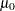Null value of test (value of H0= option in PROC TTEST statement)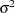Population variance
Population value of standard deviation
Population value of geometric mean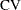Population value of coefficient of variation (ratio of population standard deviation and population arithmetic mean)Value of ALPHA= option in PROC TTEST statement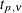th percentile ofdistribution with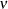degrees of freedom (d.f.)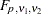th percentile ofdistribution with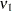numerator d.f. and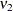denominator d.f.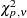th percentile of chi-square distribution withd.f.